Home > A2C > Chapter 9 > Lesson 9.3.2 > Problem9-163

9-163.
1. For homework, Londa was asked to simplify the expression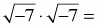. She got the answer 7, but when she checked, she learned that the correct answer was −7.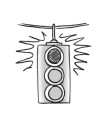Homework Help ✎

1. Show Londa the steps she could take to get −7.

2. What steps do you think Londa took to get 7 as a result?

3. What does she need to consider in order to avoid making this mistake in the future?

4. Londa's example means that it is not always true that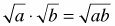for real numbers a and b. What restriction needs to be placed on the numbers a and b?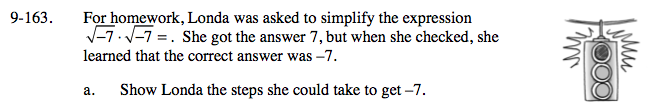First eliminate the negative sign from each radical by replacing it with i.

Remember that i is the square root of −1, now finish multiplying.

−7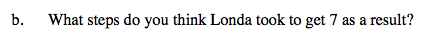$\text{Londa multiplied }\sqrt{-7}\cdot\sqrt{-7}\text{ to get }\sqrt{49} = 7$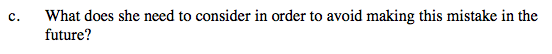See parts (a) and (b).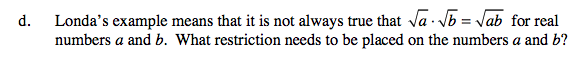The variables a and b must be non-negative real numbers.